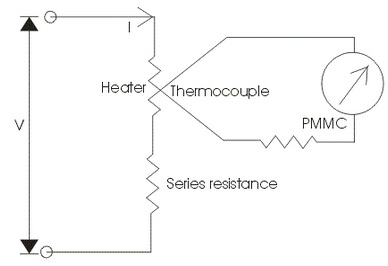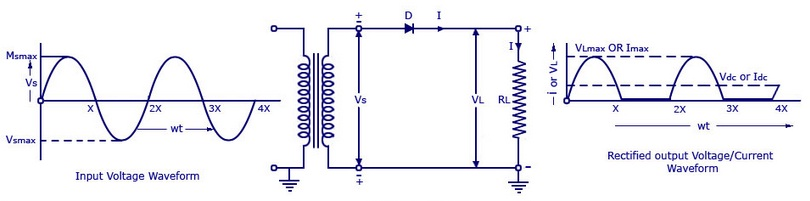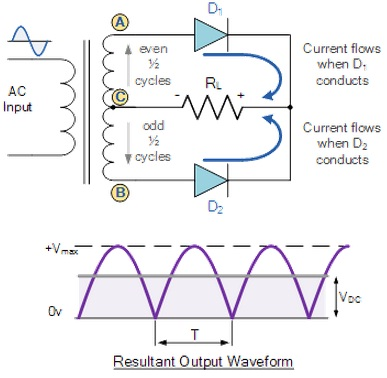#### Alternating Current Theory, Physics tutorial

Alternating currents and Voltage:

The effects of a.c are necessarily similar as those of d.c. Both are suitable for heating and lighting purposes. As we have observed, a.c. is more simply produced and distributed than d.c and for this reasons the main supply is a.c.

Definition of Alternating Current or Voltage:

The alternating current or e.m.f differs periodically by time in magnitude and direction. One complete alternation is termed as a cycle and the number of cycles taking place in one second is known as the frequency (f) of the alternating quantity (that is, current or voltage).

The unit of frequency is the hertz (Hz) and was formerly the cycle per second (c.p.s).

In general, f = 1/T.

The simplest and most significant alternating e.m.f. can be symbolized through a sine curve and is stated to have a sinusoidal wave form. It can be represented by the equation:

E = Eo sin wt

Here 'E' is the e.m.f at time t, Eo is the peak or maximum e.m.f and 'W' is a constant that equivalents 2πf where 'f' is the frequency of the e.m.f. likewise, for a sinusoidal alternating current, we have:

I = Io sin wt

In the above equation, 'I' is the instantaneous value of the current at a given instant 't' and Io its maximum or peak value or the present amplitude.

Effective Value of an Alternating Current or Voltage:

Is it possible to measure the alternating current by means of an ordinary D.C. ammeter? The answer is No. This is due to the reason that throughout one half cycle of the current the pointer will move in one direction and throughout the other half cycle, to the similar extent in the opposite direction. All this movement on either side will be so fast that the pointer will appear to be stationary at zero that is the average value of the current over a complete cycle.

Average current = (oT Idt)/(oT dt) = ∫TIo sinwt/T = 0

The similar will be the case when we wish to compute an alternating potential difference by means of an ordinary d.c. voltmeter. This too will represent zero deflection of the pointer, pointing to zero average value of the alternating potential difference over a whole cycle.

Thus, in the case of alternating current and potential difference we take the average over half a cycle or half a time period.

Iavg (T/2) = oT/2 Idt/oT/2 dt = oT/2 [Iosinwt dt/(T/2)] = 2Io/π

(As T = 2π/W)

Likewise, average potential difference, V = 2Vo

The average value of the alternating current or Potential difference is apparently similar for either half of a cycle however opposite in direction. For a whole cycle, thus, it follows that the average is zero.

You might then ask at this junction, how do we compute an alternating current?

The answer is that we must base our measuring tool on some such effect of the current that is independent of its direction (or which based on the square of the current, that is, I2, in such a way that there will be no negative value of I2).

One of such effects is the heating effect of the current. For, however the current flows in one direction in a resistance throughout one half cycles and in the opposite direction throughout the other half, the later doesn't reverse the heating effect generated by the former however itself generates an equivalent heating effect. Therefore a heating effect is generated by the cycle or the alternating current as a whole. We thus, state an alternating current in terms of a direct or steady current that generates the similar heating effect as the given alternating current.

The effective value of an alternating current is stated as that value of a direct current that generates the similar heating effect in a given resistance as the alternating current.

Now, heat generated by a direct current 'I' in a resistance 'R' in time 'r is equivalent to I2Rt. Let the similar heating effect be generated in the similar resistance R in time t through an alternating current 'I'.

Ieff = Io/√2

The equation above represents that the effective value of an alternating current is 1/2 times its maximum or peak value. The effective value is as well termed as the root mean square (r.m.s.) value of the current.

Effective value of p.d. Veff   = Vo/√2

Alternating Current Meters:

We are familiar that the deflection of an a.c. meter should not base on the direction of the current. Most of the voltmeters and ammeters for a.c. use are calibrated to read r.m.s values and presents right readings only when the waveform is sinusoidal.

Moving-Iron Meter:

This machine or instrument is one of the most primitive forms of measuring and relay instrument. Moving iron kind instruments are of basically two kinds. Attraction type and repulsion type instrument.

If a piece of iron is positioned closer to a magnet it would be attracted by the magnet. The force of this attraction based on the strength state magnetic field. If the magnet is electromagnet then the magnetic field strength can simply be raised or reduced through increasing or decreasing current through its coil. Accordingly the attraction force acting on the piece of iron would as well be increased and decreased. Based on this simple phenomenon attraction type moving iron instrument was introduced.

If the two pieces of iron are kept side by side and a magnet is brought closer to them the iron pieces will repulse one other. This repulsion force is due similar magnetic poles induced in the similar sides the iron pieces due to the external magnetic field. This repulsion force rises if field strength of the magnet is increased. Similar case, when the magnet is electromagnet, then magnetic field strength can simply    be controlled through controlling input current to the magnet. Therefore whenever the current raises the repulsion force among the pieces of iron is raised and if the current reduces the repulsion force between them is reduced. Based on this phenomenon repulsion kind moving iron instrument was constructed.

Thermocouple Meter:Widely speaking the thermocouple kind of instruments comprises of two main portions which are written below:

Thermo electric elements: The thermocouple type of instruments consists of thermo electric elements which can be of four types:

1) Contact type: It consists of a separate heater that is represented in the diagram above. The action of thermocouple kind instruments can be described briefly as,

A) At junction, the electrical energy is being transformed to thermal energy in the heater element. A part of the heat is transferred to the hot junction whereas most of the heat energy is dissipated away.

B) The heat energy that is transferred to hot junction is again transformed to electrical due to Seebeck effect. Just a part of electrical energy is transformed into mechanical energy that is employed to generate a deflecting torque. The total efficiency of the system is low therefore the instrument consumes high power. Therefore there is a requirement of highly accurate and sensitive dc instrument.

2) Non contact type: In the non contact type there is insulation among the heating element and the thermocouple that is, there no direct contact between the two. Due to this such instruments are not much responsive as compared to contact type.

3) Vacuum thermo-elements: Such kinds of instruments are mainly employed for the measurement of electric current at extremely high frequency of the order of 100 Mega hertz or more as such instruments keep their accuracy even at such high frequency.

4) Bridge type: Such bridges are formed on the ac ratings generally from 100 milli amperes to 1 amperes. In such two thermocouples are joined to form a bridge.

Rectifier Meter:

Rectifier kind tool computes the alternating voltage and current by the help of rectifying elements and permanent magnet moving coil type of tools. Though the primary function of rectifier kind of tools work as the voltmeter.

Now the question occurs in our mind why we make use of rectifier kind of instruments broadly in the industrial world however we have different other ac voltmeter similar to electrodynamometer kind instruments, thermocouple type instruments and so on? The answer to this question is as follows:

1) The cost of electrodynamometer type of instruments is quite high than rectifier type of tools. However the rectifier type of instruments as much precise as electrodynamometer kind of instruments. Therefore rectifier type of instruments is favored over electrodynamometer type instruments.

2) The thermocouple tools are more fragile than the rectifier kind of instruments. Though thermocouple type of instruments is much broadly employed at much high frequency.

Long Distance Power Transmission:

We have observed that the principle of electromagnetic induction makes alternating current preferable to direct current for big-scale production of electricity.

Electric power has to be transmitted to towns and villages from power stations, generally positioned at fairly distant places where better and cheaper facilities for power generation are available. Long cables, termed as transmission lines are employed. The arrangement is favored to as a Grid system.

We should have noticed as we travel from one place to the other in our Local Government Area or State, that the Grid system is a network of cables (or wires), most of it supported on pylons, that joins very many power (PHCN) stations all through the country and carrying electrical energy from then to consumers.

When the current were to be transmitted at comparatively low voltage at which it is produced at the power station, the current strength would be high and would outcome in considerable loss of energy in the form of heat in the transmission lines.

Rectification of Alternating Current:

The Rectification is the conversion of a.c to d.c through a rectifier. The main sources of D.C. voltages are D.C. power supplies. They are different from batteries in that they get energy from an a.c power source. The transformation of a.c. into D.C. can be accomplished in some different ways based on the needs of D.C. power. Half-wave rectifiers, full-wave rectifiers, filtered power supplies, and regulated power supplies are used.

Rectifiers encompass a low resistance to current flow in one direction, termed as the forward direction, and a high resistance in the opposite or reverse direction. They are conductors that are mainly unidirectional.

If connection is made to a supply in such a way that a rectifier conducts, it is stated to be forward biased; and in the non-conducting state it is reverse based.

Half-wave Rectifier:A simple Half Wave Rectifier is more than a single PN-junction diode joined in series to the load resistor. In this we are providing an alternating current as input. Input voltage is given to a step down transformer and the resultant reduced output of transformer is given to the diode 'D' and load resistor 'RL'. The output voltage is computed across load resistor RL.

Working of Half Wave Rectifier:

The input given to the rectifier will encompass both negative and positive cycles. The half rectifier will let only the positive half cycles and skip the negative half cycles.

Positive Half Cycle:

In the positive half cycles if the input AC power is provided to the primary winding of the step down transformer, we will obtain the reduced voltage at the secondary winding that is given to the diode.

The diode will let current flowing in clock-wise direction from anode to cathode in the forward bias (that is, diode conduction will occur in the forward bias) that will produce just the positive half cycle of the AC.

The diode will eradicate the variations in the supply and provide the pulsating DC voltage to the load resistance RL. We can obtain the pulsating DC at the Load resistance.

Negative Half Cycle:

In negative half cycle, the current will flow in anti-clockwise direction and the diode will go in to the reverse bias. In the reverse bias the diode will not conduct so, no current in flown from anode to cathode, and we can't obtain any power at the load resistance.

Just small amount of reverse current is flown from the diode however this current is nearly negligible. And voltage across the load resistance is as well zero.

Full-Wave Rectifier:Similar to the half wave circuit, a Full Wave Rectifier Circuit generates an output voltage or current that is purely DC or consists of some specified DC component. Full wave rectifiers encompass a few basic benefits over their half wave rectifier counterparts. The average (DC) output voltage is higher than for half wave, the output of full wave rectifier has much less ripple than that of the half wave rectifier generating a smoother output waveform.

In a Full Wave Rectifier circuit two diodes are now employed, one for each and every half of the cycle. A multiple winding transformer is employed whose secondary winding is divided uniformly into two halves having a common centre tapped connection, (C). This configuration outcome in each and every diode conducting in turn if its anode terminal is positive with respect to the transformer centre point C generating an output throughout both half-cycles two times that for the half wave rectifier therefore it is 100% efficient.

The full wave rectifier circuit comprises of two power diodes joined to a single load resistance (RL) with each diode taking it in turn to supply the current to the load. If point 'A' of the transformer is positive with respect to point 'C', diode D1 conducts in the forward direction as pointed by the arrows.

If point 'B' is positive (in the negative half of the cycle) with respect to point C, diode D2 conducts in the forward direction and the current flowing via resistor 'R' is in the similar direction for both the half-cycles. As the output voltage across the resistor 'R' is the phasor sum of the two waveforms joined, this kind of full wave rectifier circuit is as well termed as 'bi-phase' circuit.

Tutorsglobe: A way to secure high grade in your curriculum (Online Tutoring)

Expand your confidence, grow study skills and improve your grades.

Since 2009, Tutorsglobe has proactively helped millions of students to get better grades in school, college or university and score well in competitive tests with live, one-on-one online tutoring.

Using an advanced developed tutoring system providing little or no wait time, the students are connected on-demand with a tutor at www.tutorsglobe.com. Students work one-on-one, in real-time with a tutor, communicating and studying using a virtual whiteboard technology.  Scientific and mathematical notation, symbols, geometric figures, graphing and freehand drawing can be rendered quickly and easily in the advanced whiteboard.

Free to know our price and packages for online physics tutoring. Chat with us or submit request at info@tutorsglobe.com